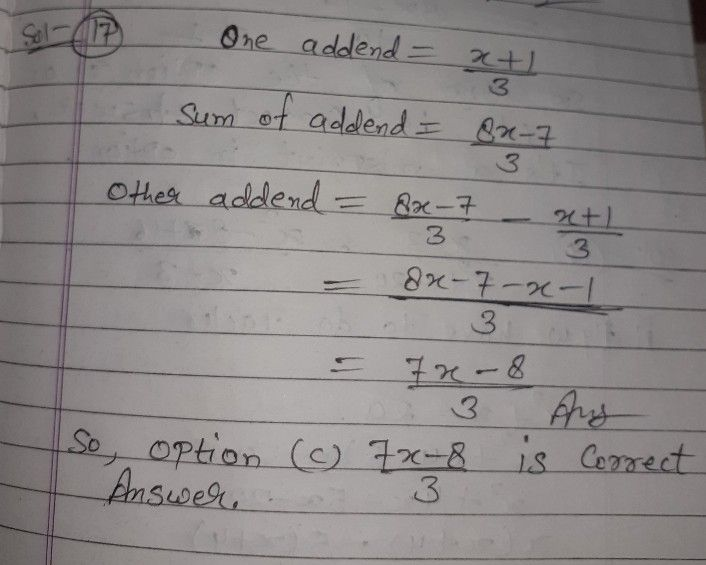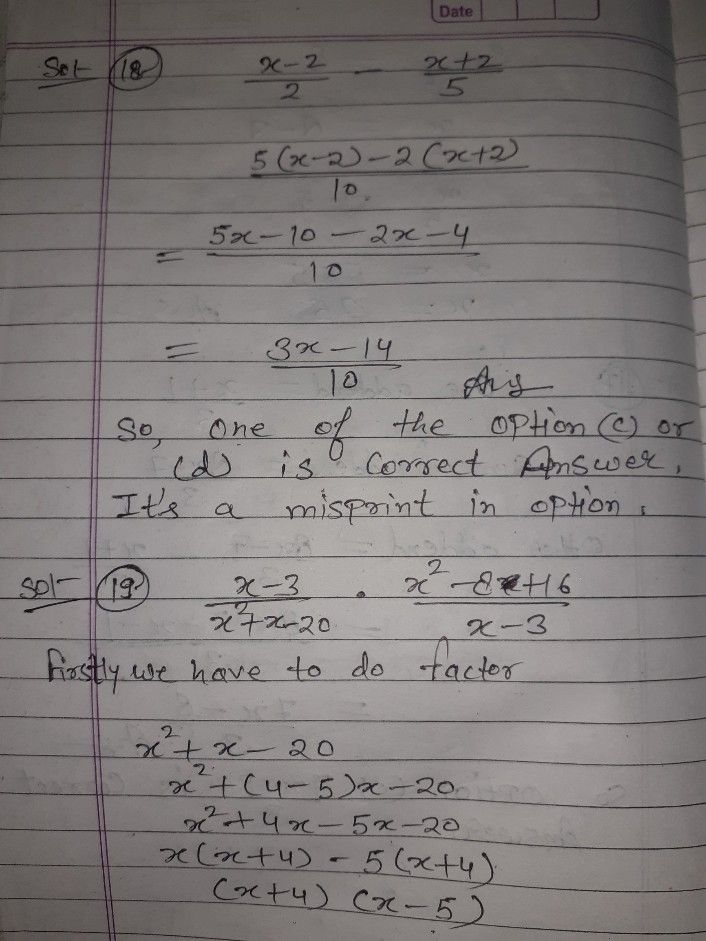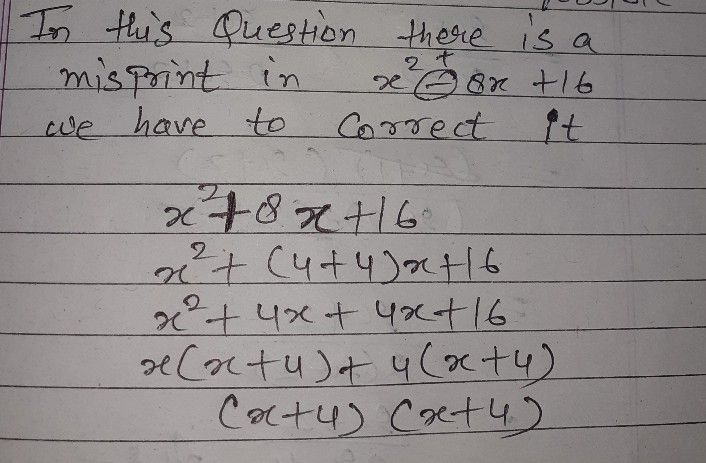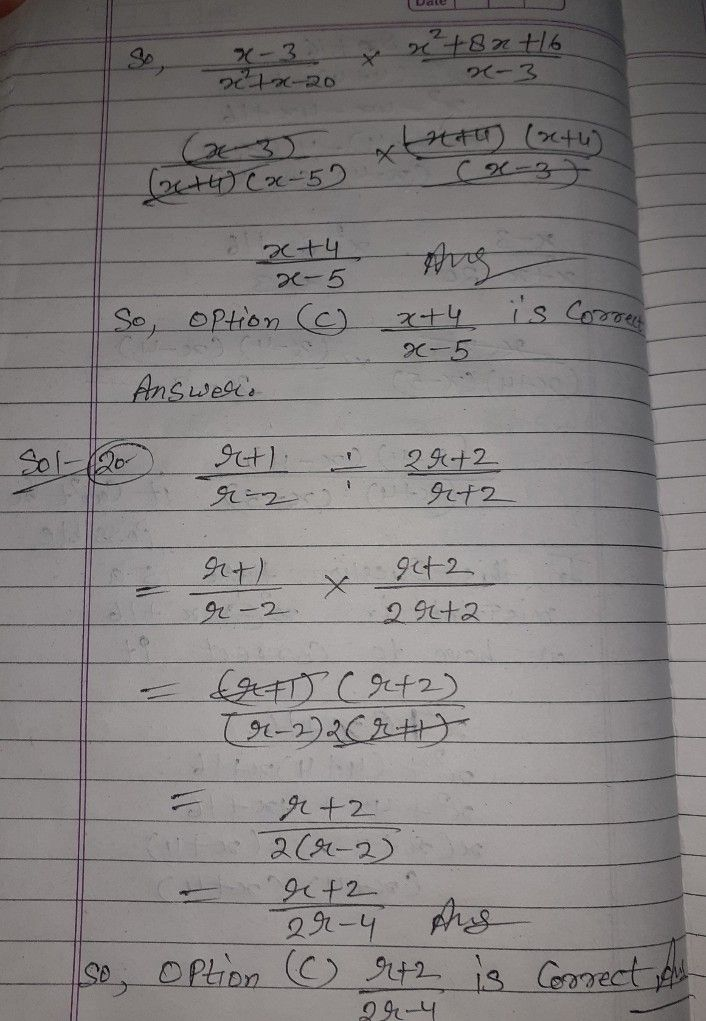Symbol
Problem$-17$ Given as one addend of the sum $\dfrac {8x-7} {3}$ find the other addend? A. 72-4 B. $\dfrac {7x-6} {3}$ C. 7x3 -8 D. $\dfrac {7x-10} {3}$ _18. Perform the indicated operation. $\dfrac {x-2} {2}-\dfrac {x+2} {5}$ $A.\dfrac {3x+6} {10}$ B. $\dfrac {3x-6} {10}$ C. $\dfrac {3x+14} {10}$ D. $\dfrac {3x+14} {10}$ 19. What is the product of $\dfrac {x-3} {x^{2}+x-20}$ $\dfrac {x^{2}-8+16} {x-3}7$ $x$ $A-$ $-$ B.7 r+5 $C.\dfrac {x+4} {x-5}$ D. *x++4 5 p. Divide $im\dfrac {2\pi +2} {-→}$ $\dfrac {2r+2} {r+2}$ $r-2$ $-$ 2T7-+1 2 B. 2r+1 $C.\dfrac {r+2} {2r-4}$ D. $\dfrac {r-2} {2r+4}$
7th-9th grade
Algebra
Search count: 106
SolutionQanda teacher - SHIVKUMARCokk
I hope you understand
If you have any problem with answer plz ask me or inform me first.
But If you're satisfied plz do like button and give 5 stars rating as a gift of Thank you..??